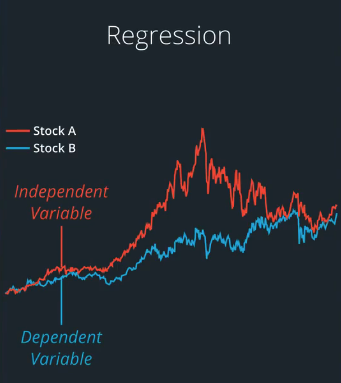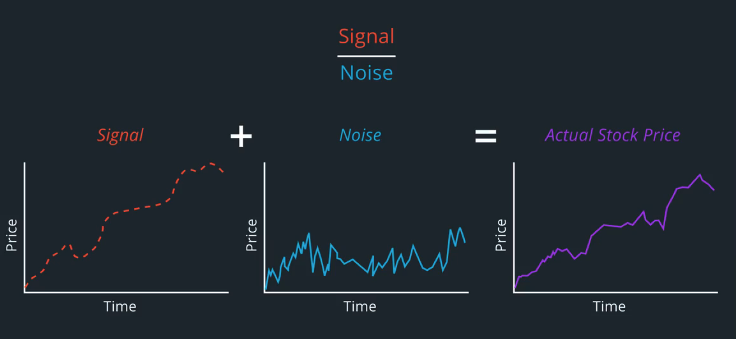# AI For Trading: Regression (15)

## Intro

### Checking and transforming dataNote: Regarding the chart used to describe signal to noise. The horizontal x-axis of the chart is time, such as days. The vertical y-axis is price, such as dollars. The blue line represents the combined signal plus noise, which is the actually observed stock price movement. The red dashed line represents the signal without noise, which is not directly observable.

## Distributions

Many statistical models assume that the data follows a normal distributions, also referred to as a Gaussian or a bell curve.

This is important when checking whether our models are valid. There are various tests that we use to check that our models describe a meaningful relationship.

## Exercise: Visualize Distributions

Many variables tend to follow a Normal distribution (hence the name “Normal”), both in nature as well as artificial contexts. But there are other distributions as well, some that are variants of the Normal distribution, and some that are completely different! Each distribution is suitable for modeling certain kinds of variables.

In this exercise, you are given some samples of data. Plot the histogram of each sample, and then try to match it with the corresponding distribution.

"""Visualize the distribution of different samples."""

import pandas as pd
import matplotlib.pyplot as plt

def plot_histogram(sample, title, bins=16, **kwargs):
"""Plot the histogram of a given sample of random values.

Parameters
----------
sample : pandas.Series
raw values to build histogram
title : str
bins : int
number of bins in the histogram
kwargs : dict
any other keyword arguments for plotting (optional)
"""
# TODO: Plot histogram (no need to return anything)
# width = 0.7 * (bins - bins)
# center = 8
# plt.bar(center, sample, align='center')
print(sample)

# plt.title(title);
plt.show()

def test_run():
"""Test run plot_histogram() with different samples."""
# Load and plot histograms of each sample
# Note: Try plotting them one by one if it's taking too long
plot_histogram(A, title="Sample A")